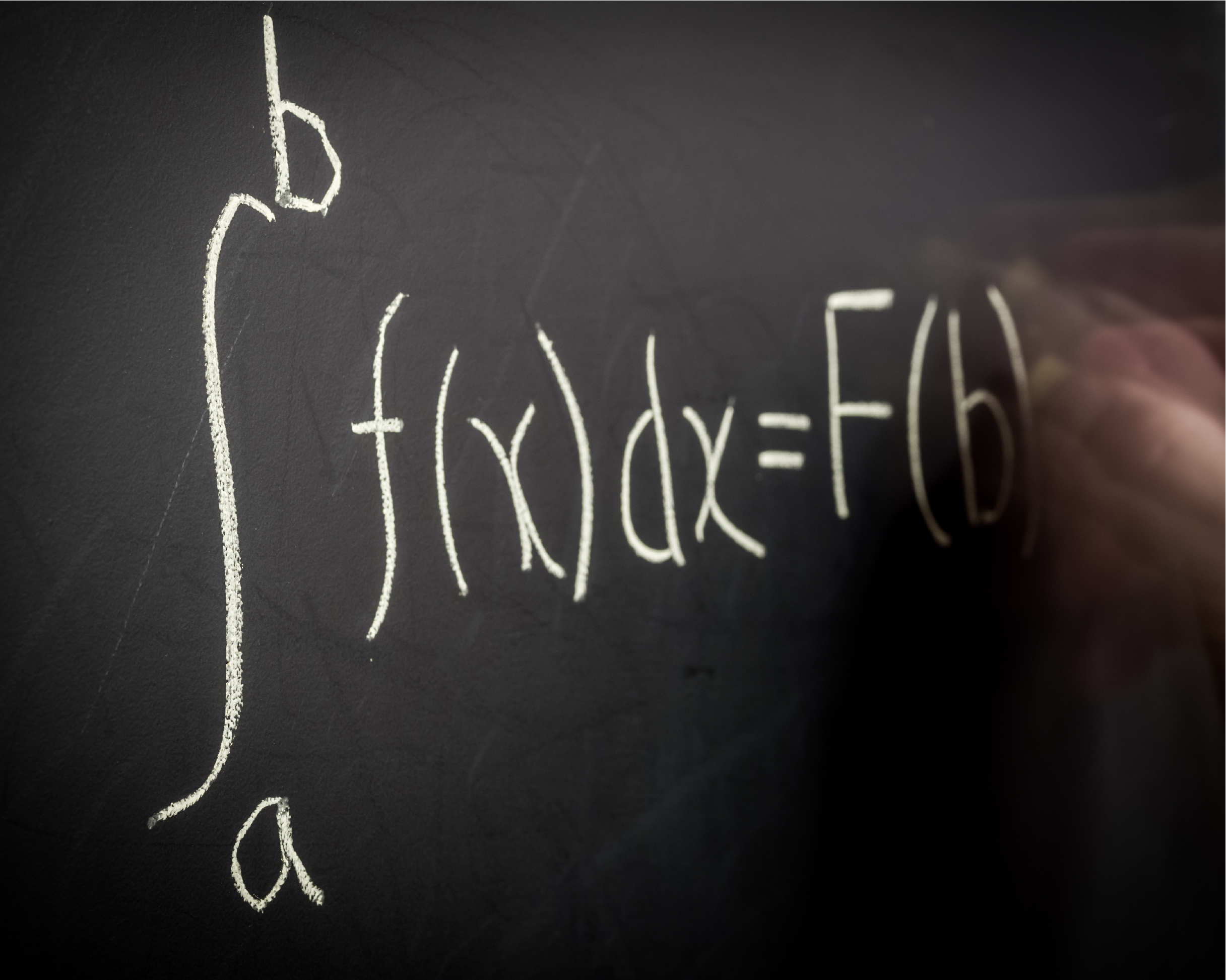Are you familiar with the Mean Value Theorem? Can you calculate derivatives? The AP® Calculus BC exam tests topics and skills discussed in your AP Calculus BC course. If you score high enough on the exam, your AP Calculus score could earn you college credit!

Check out our AP Calculus BC Guide for the essential information you need for the exam:

## What’s on the AP Calculus BC Exam?

The College Board is very detailed in what they require your AP teacher to cover in his or her AP Calculus BC course. You should be familiar with the following topics:

• Limits and Continuity—Defining limits, estimating limits from graphs and tables, determining limits using algebraic properties and manipulation, applying the Squeeze Theorem, Determining types of discontinuities, understanding asympototes, and the applying Intermediate Value Theorem

• Differentiation: Definition and Basic Rules—Defining average and instantaneous rates of change, defining the derivative of a function, estimating derivatives at a point, connecting differentiability and continuity, applying the Power Rule, the Product Rule, and the Quotient Rule, and determining derivatives of constants, sums, differences, and constant multiples, trigonometric functions, ex , and ln x

• Differentiation: Composite, Implicit, and Inverse Functions—Applying the Chain Rule, using implicit differentiation, differentiating inverse functions, and calculating higher order derivatives

• Contextual Applications of Differentiation—Interpreting derivatives in context, using rates of change in motion and other context, applying related rates, approximating using linearization, and applying L’Hospital’s Rule

• Analytical Applications of Differentiation—Understanding the Mean Value Theorem, using the Extreme Value Theorem, finding global and local extrema, applying the First Derivative Test and Second Derivative Test, finding intervals of increase and decrease, understanding concavity, sketching graphs, solving optimization problems, and using implicit relations

• Integration and Accumulation of Change—Finding accumulations of change, Reimann sums, and definite integrals, understanding the Fundamental Theorem of Calculus, interpreting accumulation functions, finding anti-derivatives and indefinite integrals, and integrating using substitutions, long division, completing the square, integration by parts, linear partial fractions, and improper integrals

• Differential Equations—Modeling situations with differential equations, verifying solutions for differential equations, sketching slope fields, approximating using Euler’s Method, and using separation of variables

• Applications of Integration—Finding the average value of a function, connecting position, velocity, and acceleration using integrals, applying accumulation functions, finding area between curves of functions, finding volumes from cross-sections and revolutions, and finding arc length

• Parametric Equations, Polar Coordinates, and Vector-Valued Functions—Defining and differentiation parametric equations, finding second derivatives of parametric equations, finding arc lengths of parametric equations, defining and differentiating and integrating vector-valued functions, solving motion problems using parametric and vector-valued functions, defining polar coordinates, differentiating polar functions, finding the area of regions bounded by a single polar curves or two polar curves

• Infinite Sequences and Series—Defining convergent and divergent infinite series, working with geometric series, determining convergence and divergence using the n th Term Test, Integral Test, Comparison Test, Alternative Series Test, and Ratio Test, working with geometric series and p-series, determining absolute or conditional convergence, determining error bound, finding Taylor polynomials, finding Lagrange error bound, finding radius and interval of convergence of power series, and representing functions as power series

## AP Calculus AB vs. BC

AP Calculus is divided into two classes: AB and BC. The College Board says Calculus AB is the equivalent of a semester of college calculus and BC is the equivalent of a year of college calculus. In truth, AB calculus covers closer to three quarters of a year of college calculus. The main difference between the two is that BC Calculus tests some more theoretical aspects of calculus, and it covers a few additional topics.

## AP Calculus BC Sections and Question Types

The AP Calculus BC exam is three hours long and has two sections: a multiple-choice section and a free-response section. Each exam section has a Part A and a Part B.

 Section Timing Number of Questions % of Exam Score Section 1 Part A: 60 minutes Part B: 45 minutes Part A: 30 multiple choice questions Calculator not permitted Part B: 15 multiple choice questions Calculator permitted 50% Section 2 Part A: 30 minutes Part B: 60 minutes Part A: 2 free response questions Calculator permitted Part B: 4 free response questions Calculator not permitted 50%

### Multiple-Choice

The multiple-choice questions on the AP Calculus BC exam cover a variety of calculus topic are discrete, as opposed to appearing in question sets, and will have a similar format that is followed by five answer choices.  At times, it may seem that there could be more than one possible correct answer. Answers resulting from common mistakes are often included in the five answer choices to trap you.

### Free Response Questions

The FRQ section of the AP Calculus BC exam consists of six questions that require you to write out the solutions and steps by which you solved it. Partial credit is given for various steps in the solution of each problem. You’ll usually be required to sketch a graph in one of the questions.

## AP Calculus BC Scores

AP scores are reported from 1 to 5. Colleges are generally looking for a 4 or 5 on the AP Calculus BC exam, but some may grant credit for a 3. Learn more about college AP credit policies. Each test is curved so scores vary from year to year. Here’s how AP Calculus BC students scored on the May 2020 test:

 Score Meaning Percentage of Test Takers 5 Extremely qualified 44.6% 4 Well qualified 17.6% 3 Qualified 19.4% 2 Possibly qualified 14.1% 1 No recommendation 4.3%

Source: College Board

## How can I prepare?

AP classes are great, but for many students they’re not enough! For a thorough review of AP Calculus BC content and strategy, pick the AP prep option that works best for your goals and learning style.